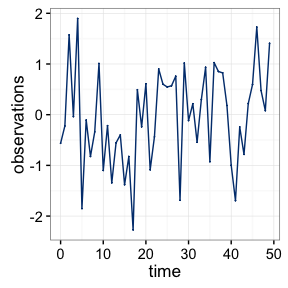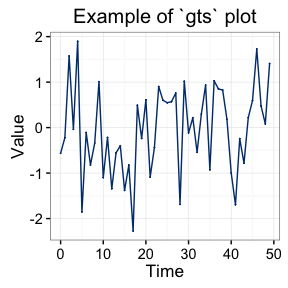# Introduction

The objective of this document is to provide users background information on the supported data objects within the gmwm ecosystem. The document provides information on how the different data types to use when loading a data set, how to create a model statement, and how to work with the computational results.

# Data Storage Objects

This section details the different kinds of objects recognized to store ones data. The section breaksdown the object and the benefits of using it.

## gts object

The gts object is the gmwm package’s equivalent to the ts object in R. There are a few differences between the two objects. Most notably, the unit conversion ability of the gts object. Furthermore, the gts object’s plotting functionality uses ggplot2 instead of the base plotting library making cleaner graphs.

### Loading data into a gts

To populate a gts object there are two options:

vals = rnorm(100)

# Basic
ex1.basic = gts(vals)

ex1.adv = gts(vals, freq = 2, unit = "sec", name = "Example 1 Data")
1. Generate data according to an underlying process
N = 1000
model = WN(sigma2=1) + AR1(phi = 0.1, sigma2 = .7)

# Basic
ex2.basic = gen.gts(model, N)

ex2.adv = gen.gts(model, freq = 10, unit = "sec", name = "Example 2 Data")

Within these data load options, you can supply additional information to generate an advanced gts object:

1. freq: The frequency of the data
• We assume that the frequency of an object is 1.
2. unit: The unit the data was written in.
• By default, no unit is associated with your data.
3. name: A name to be associated with your dataset
• Data sets without a name will have it autogenerated within the plot function as "Data Set X", where "X" denotes a number

### Working with gts

To use the gts object in your own code, there are a few helpful functions:

1. Detect whether or not the object is gts
is.gts(x)
1. Find out the length of the time series
length(x)
nrow(x)

### Graphing with gts

To create a graph with the gts object use:

x = gen.gts(WN(sigma2=1), 50)
plot(x)This can be improved by doing:

plot(x, title = "Example of gts plot", axis.x.label = "Time", axis.y.label = "Value")## imu object

The imu object is meant to act as a means to provide structure to an inertial measurement unit (IMU) data set. Using this structure enables an automatical modeling selection procedure through auto. Furthermore, we are able to provide a more customized visual graph for each of the sensors.

### Loading data into an imu

To populate an imu object there are two options:

N = 10000
vals = cbind(rnorm(N),
rnorm(N, mean = 3, sd = 1),
rnorm(N, mean = 2, sd = 3),
rnorm(N, mean = 1, sd = 2))

# Basic
x = imu(vals,
gyros = 1:2,
accels = 3:4)

x = imu(vals,
gyros = 1:2,
accels = 3:4,
axis = c("A","B"),
freq = 5, unit = "sec", name = "Example 3 Data")
1. Load in an IMU binary file
# Option 1: Relative Directory
setwd("C:/Users/James")
x = read.imu("Documents/imu_data.imu", type = "IXSEA")

# Option 2: Fixed Directory
x = read.imu("C:/Users/James/Documents/imu_data.imu", type = "IXSEA")

The binary file reader currently supports the binary record format accepted by well known commercial software from Applanix (PosProc - developed by Bruno Scherzinger) or Novatel/Waypoint (IExplorer). This format is aptly described as a seven column layout where the first column of the data contains time data, columns 2-4 contain gyroscope values and columns 5-7 contain accelerometer values for axes X, Y, and Z. On load, appropriate scaling factors are applied to the data. This input format is valid for the following IMUs:

• IMAR
• LN200
• LN200IG
• IXSEA
• NAVCHIP_INT
• NAVCHIP_FLT

As was the case with the gts object, you can supply additional information to generate an advanced imu object such as:

1. freq: The frequency of the data
• We assume that the frequency of an object is 1.
2. unit: The unit the data was written in.
• By default, no unit is associated with your data.
3. name: A name to be associated with your dataset
• Data sets without a name will have it autogenerated within the plot function as "Data Set X", where "X" denotes a number

### Working with imu

To use the imu object in your own code, there are a few helpful functions:

1. Detect whether or not the object is imu
is.imu(x)
1. Find out the length of the group of time series
nrow(x)
1. Find out the number of columns
ncol(x)
length(x)
1. Find out the sensors in the imu object
# Numeric Return
value(x,"accel")   # Number of Accelerometers
value(x,"gyro")    # Number of Gyroscopes
value(x,"sensors") # Total Number of Sensors

# Logical Return (T/F)
has(x, "accel")
has(x, "gyro")
has(x, "sensors")
1. View n observations at the start or end of the data set
# Start of data set

# End of data set
tail(x, 5)

## lts object

The lts object represents data from a latent time series. This object is meant to act as a means to demo the decomposition of a state-space model. If you do not need to prepare a demo, it is not advised to use this function as it provides a more detailed representation of the processes.

## Implementation of Base Objects

While the gmwm package does provide its own data object types, we do realize that you may wish to use R’s base objects. The following R base objects are supported within the computational framework: ts, matrix, and data.frame.

# Supplying Models

For the package, we’ve opted to create a new method for supplying Time Series models. We feel that this framework is more natural than methods that presently exists within the R ecosystem. Using the ts.model S3 object we’ve created will provide considerably flexibility in employing a large variety of processes.

## Supported Processes

The ts.model object currently supports the following time series processes:

• Autoregressive order 1 (AR1(phi,sigma2))
• Gauss-Markov Order 1 (GM(beta,sigma2_gm))
• Normal White Noise (WN(sigma2))
• Random Walk (RW(sigma2))
• Quantization Noise (QN(q2))
• Moving Averages of order Q (MA(q))
• Autoregressive of order P (AR(p))
• Autoregressive - Moving Averages of orders P,Q (ARMA(p,q,sigma2))

Please note, that we assume the frequency of an object is 1. Thus, a GM() term without a freq set is equivalent to an AR1() term. By changing the frequency within the gts or imu object, the interpretation of the GM() term will change whereas the AR1() term will not.

## Specifying Processes

One key feature of the ts.model object is that it enables users to specify models with initial starting parameters or let the built in grid search algorithm guess initial parameter values.

# Creates an AR1 modeling component with the program set to guess initial values.
model.guided = AR1()

# Makes an AR1 modeling component with user supplied initial values.
model.adv = AR1(phi = .3, sigma2 = 1)

## Chaining Processes

In the prior section, we showed how to use the modeling syntax to model just one process. Here we expand this approach to model multiple processes by chaining different modeling terms together through the use of the plus operator.

# Creates an AR1 + WN model with the program set to guess initial values.
model.guided = AR1() + WN()

# Builds an AR1 + WN model with user supplied initial values.
model.adv = AR1(phi = .9, sigma2 = 1) + WN(sigma2 = .1)

# Creates an ARMA(2,2) Process
arma.guided = ARMA(2,2)

# Specifying parameters for an arma 2,2
arma.adv = ARMA(ar = c(0.3,.7), ma = c(0.5,.1), sigma2 = 1)

It is important to note that you are NOT able to mix supplied values with program guessed values. Thus, at the this moment in time, a mixed model given by AR1() + WN(sigma2 = 1) will default to guessing all parameters. Therefore, the model statement must either have supplied initial values for each term or provide a number of parameters and be empty.

For more complex models, where the program is set to guess the initial values, you can simplify the model by using multiplication.

# Creates an 3AR1 model with the program set to guess initial values.
model.rep = 3*AR1()

# Equivalent to:
model.add = AR1() + AR1() + AR1()

There are a few limits to chaining. Specifically, we can only recover one of each of the following model components: DR(),QN(),RW(),WN(). This means that DR() + QN() + RW() + WN() is a valid model but 2*DR() is NOT a valid model.